#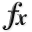N-D Test Functions T¶

class go_benchmark.TestTubeHolder(dimensions=2)

TestTubeHolder test objective function.

This class defines the TestTubeHolder global optimization problem. This is a multimodal minimization problem defined as follows: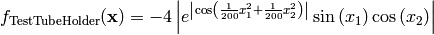Here,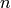represents the number of dimensions andfor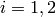.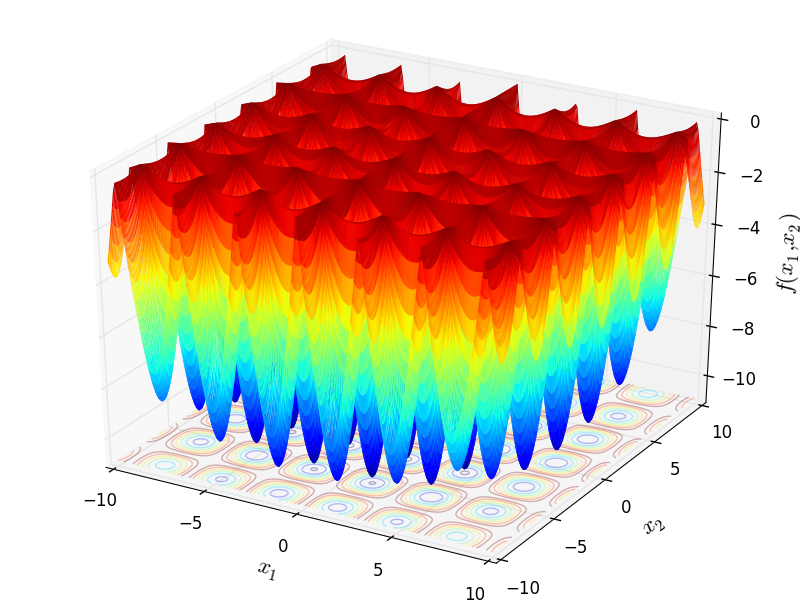Two-dimensional TestTubeHolder function

Global optimum: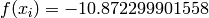forclass go_benchmark.ThreeHumpCamel(dimensions=2)

Three Hump Camel test objective function.

This class defines the Three Hump Camel global optimization problem. This is a multimodal minimization problem defined as follows: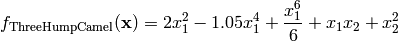Here,represents the number of dimensions andfor.Two-dimensional Three Hump Camel function

Global optimum: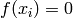forclass go_benchmark.Treccani(dimensions=2)

Treccani test objective function.

This class defines the Treccani global optimization problem. This is a multimodal minimization problem defined as follows: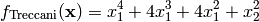Here,represents the number of dimensions andfor.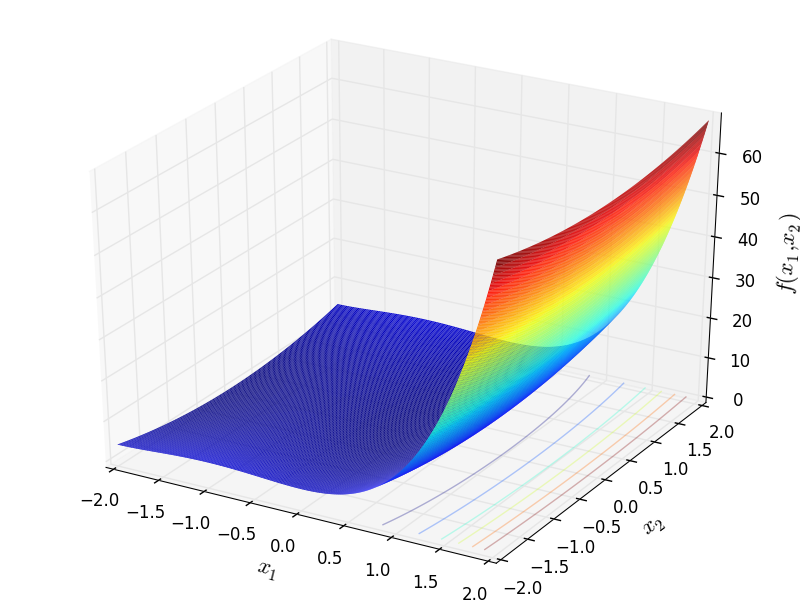Two-dimensional Treccani function

Global optimum:for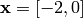or.

class go_benchmark.Trefethen(dimensions=2)

Trefethen test objective function.

This class defines the Trefethen global optimization problem. This is a multimodal minimization problem defined as follows: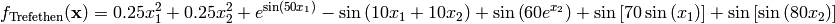Here,represents the number of dimensions andfor.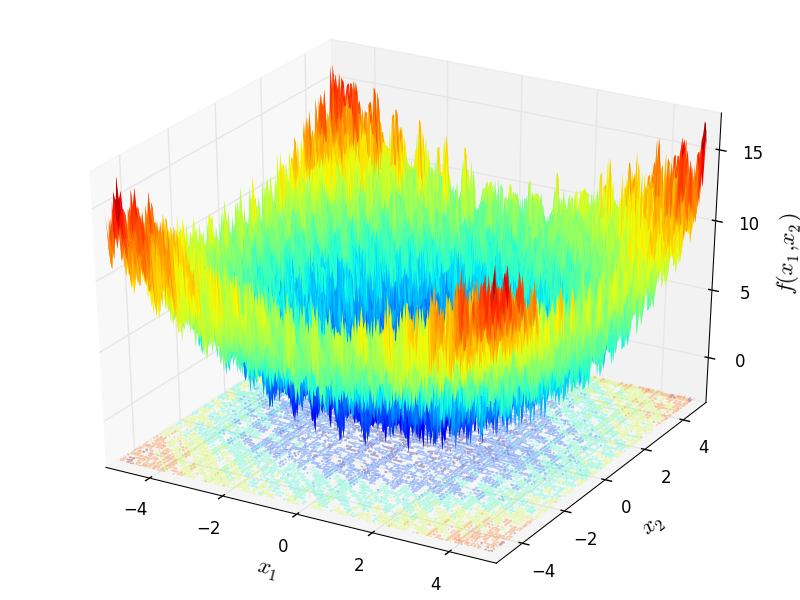Two-dimensional Trefethen function

Global optimum: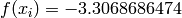forclass go_benchmark.Trid(dimensions=6)

Trid test objective function.

This class defines the Trid global optimization problem. This is a multimodal minimization problem defined as follows: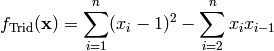Here,represents the number of dimensions and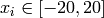for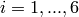.

Global optimum:for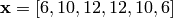class go_benchmark.Trigonometric01(dimensions=2)

Trigonometric 1 test objective function.

This class defines the Trigonometric 1 global optimization problem. This is a multimodal minimization problem defined as follows:Here,represents the number of dimensions and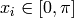for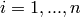.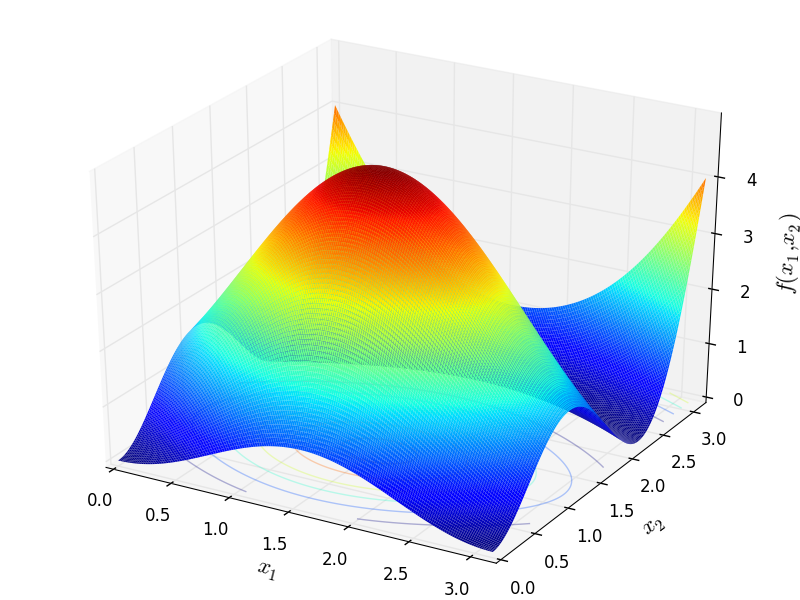Two-dimensional Trigonometric 1 function

Global optimum:for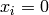forclass go_benchmark.Trigonometric02(dimensions=2)

Trigonometric 2 test objective function.

This class defines the Trigonometric 2 global optimization problem. This is a multimodal minimization problem defined as follows:Here,represents the number of dimensions and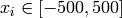for.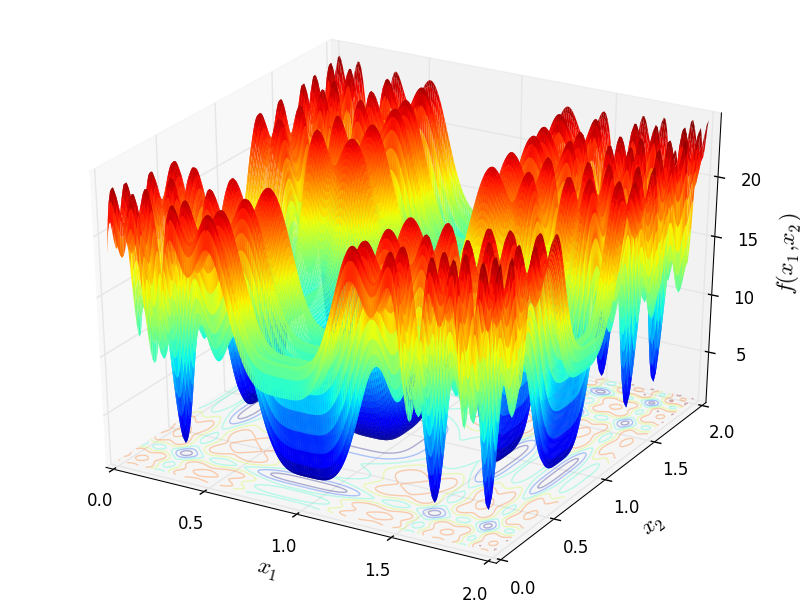Two-dimensional Trigonometric 2 function

Global optimum: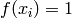forforclass go_benchmark.Tripod(dimensions=2)

Tripod test objective function.

This class defines the Tripod global optimization problem. This is a multimodal minimization problem defined as follows:Here,represents the number of dimensions and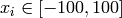for.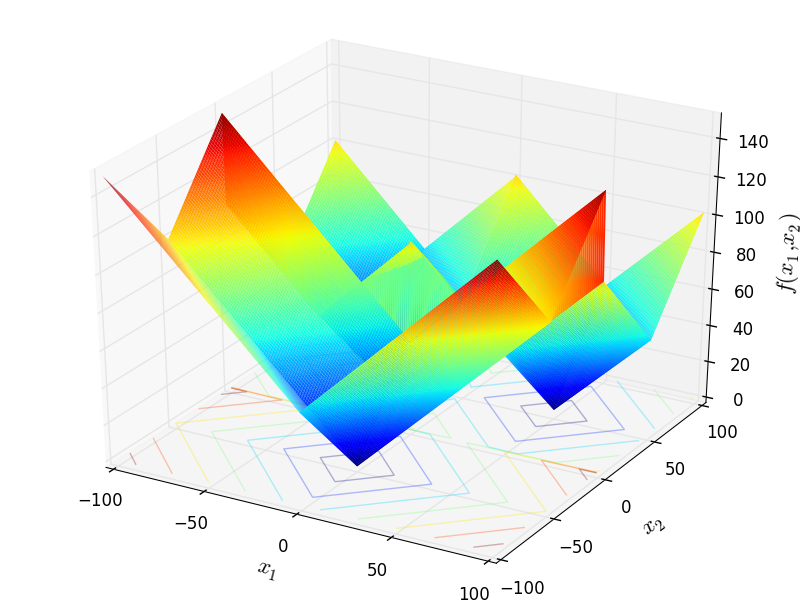Two-dimensional Tripod function

Global optimum:for#### Previous topic

N-D Test Functions S

#### Next topic

N-D Test Functions U